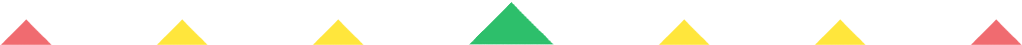Player 1
vs
Player 2
0:00
Opponent history
x
xCorrect
0
Wrong
0
Help Sheet for

• 0 + 5 = 5
• 1 + 5 = 6
• 2 + 5 = 7
• 3 + 5 = 8
• 4 + 5 = 9
• 5 + 5 = 10
• 6 + 5 = 11
• 7 + 5 = 12